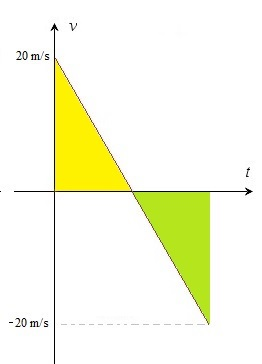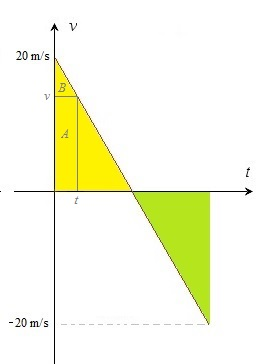# Free fall – calculating the time

Up a level : Pre DP, Mechanics and Electronics
Previous page : To throw a ball - an animation
Next page : Acceleration - calculating the timeWe will continue with the rounded value of the acceleration of gravity on the Earth as a=g=10 m/s2.

We can find the height at which an thrown object is at by using a v/t-diagram, as we saw on the precious page, or by using the formula we found on the previous pages. To recapitulate, how far has a dropped object fallen after say 3.0 seconds?

If we choose up to be in the positive direction, then a v/t-diagram would  look something like this:The velocity after 3 s is  –10·3= –30 m/s, so the speed is 30 m/s. The area is now 3·30/2=45 m.  If we use the velocity we get –45 m. This because we choose up as the positive direction.

This could, for example,  correspond to a fall from 0 m and 45 m down, or from a hight of 45 m down to the ground.

We could of course us the formula$s = \frac{{a{t^2}}}{2}$

This wound give us s= –10·32/2= –10·9/2= –90/2= –45 m as before.

Time from the displacement

Yet again, let us say we start at 0 m/s, and we ask how long time it would take to go 45 m? We start with the formula above and make the t the subject. This would give us$t = \sqrt {\frac{{2s}}{a}}$

Substituting in our values will give us$t = \sqrt {\frac{{2s}}{a}} = \sqrt {\frac{{2 \cdot 45}}{{10}}} = \sqrt {\frac{{90}}{{10}}} = \sqrt 9 = 3.0{\text{ s}}$

As expected we get the previous value back.

But an equation of the type$s = \frac{{a{t^2}}}{2}$

can have two solutions, we have ****$t = \pm \sqrt {\frac{{2s}}{a}} = \pm \sqrt {\frac{{2 \cdot ( - 45)}}{{ - 10}}} = \pm \sqrt {\frac{{ - 90}}{{ - 10}}} = \pm \sqrt 9 = \pm 3.0{\text{ s}}$

So does the time of –3 s have any physical meaning? Let us extend the v/t-diagram to negative times.  This gives usThe area to the left is as big as the area to the left, but positive. The time will now represent the time the object has to be thrown upwards to reach 45 m at the time 0 s, then to fall back down 45 m over the next 3 s.

An old example revisited

Let us look at the example from the first page about  free fall.  In that example we threw a ball straight up in the air  with the velocity 20 m/s.  An we got this graph:We start at 20 m/s and decelerate at a rate 10 m/s2.  This would give us the equation$v = 20 - 10t$

I.e. an equation of a straight line with the “y“-intercept 20 and the slope or gradient  –10.

To get a formula for the distance we can subdivide the area in a triangle and a rectangle.At the time t the area A is vt and the area B is (20-v)t/2. since v=20-10t we get the equation